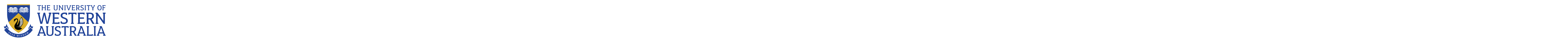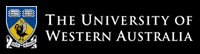Centre for Applied Statistics

# Centre for Applied Statistics

## ANOVA, Linear Regression and Logistic Regression

### Further information3 days

#### Date and Time

• 12-14 July 2021
• 6-8 December 2021
Course will run from 8:30am - 4pm all days. Registration will open at 8.15am on the first day.

#### Terms and conditions

The course is designed for people with knowledge of basic statistics who want to learn more about regression and analysis of variance (ANOVA). Please 'Enrol Now' as an expression of interest.

### Workshop Description

This course covers techniques that can be used to analyse data with continuous and categorical variables. The course will begin with simple linear regression and then proceed to approaches that can be used with more than two variables such as multiple regression. ANOVA with interactions and blocking will also be covered. The course will end with techniques that address the analysis of binary or ordinal variables.

Some basic familiarity with R is assumed (either taking An Introduction to R or Introductory Statistics in R or similar level of understanding)

### Outline

• Day 1: Simple linear regression, multiple regression, polynomial regression, regression model diagnostics, model selection.
• Day 2: One way ANOVA, blocking, simple interactions, more complex interactions, analysis of covariance, ANOVA model diagnostics.
• Day 3: Introduction to logistic regression, odds and risk ratios, multiple logistic regression, model building in logistic regression, assessing goodness of fit and model diagnostics, ordinal logistic regression.

### Cost

\$198 for those enrolled in a postgraduate research course (i.e. a Masters or PhD course eligible for Research Training Program funding) at UWA (GST inclusive)

\$845 for all other enrolments (GST inclusive)

# The University of Western Australia

## University information

CRICOS Code: 00126G